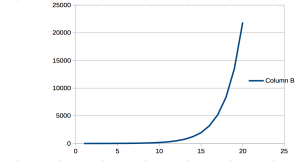A function is deemed recursive if it satisfies the following three rules:

1. There must be at least one halting condition.
2. The function must call itself.
3. The parameters thereof must be altered such that the halting condition will eventually stop the recursion.

The easiest example of a recursive function would be calculating the factorial of a (natural) number.

int fact(int n)
{
if (n == 0) /* 1. Halting condition */
return 1;
else
return n * fact ( n - 1 ); /* 2. Recursive call, 3. alteration of the parameter */;
}

As there is only a single recursive call, the recursive function will call itself a total of n times. Therefore its complexity is.

A more interesting example is an algorithm for calculating the nth Fibonacci number.

int fib(int n)
{
if( n == 0 )
return 0;
else if( n == 1 )
return 1;
else
return fib( n - 1 ) + fib( n - 2 );
}

Now there is a total of two recursive calls with two halting conditions (also called the seed values of the iteration). Noticeable is the curious amount of calls it takes for one recursion to end. If we graph the amount of calls vs. n we find a graph looking similar to an exponential function.As we now assume that the function determining the amount of calls is exponential, and that the amount of calls of n is equal to the amount of calls of n-1 plus the amount of calls of n-2. We can now, by induction, find the function mathematically.

LetSince we assume the function to be exponential we can substitute:Dividing byleaves us withSolving, we find, also called the Golden ratio. The second solution can be discarded as it is negative (approaches zero as n approaches infinity). Thus, the computational complexity of the Fibonacci function happens to be. But as there is bias to powers of two in computer science the complexity may be formally declared.

Another method of determining the computational complexity would have been sheer analysis. The iterations of a function exponentially increase by the amount of recursive calls. As the Fibonacci function calls itself two times per iteration, the analysed complexity would be, which is roughly equal to our non-rounded solution.